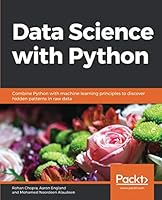# Data Science with Python## Book Description

Leverage the power of the data science libraries and advanced machine learning techniques to analyse large unstructured datasets and predict the occurrence of a particular future event.

#### Key Features

• Explore the depths of data science, from data collection through to visualization
• Learn pandas, scikit-learn, and Matplotlib in detail
• Study various data science algorithms using real-world datasets

#### Book Description

Data Science with Python begins by introducing you to data science and teaches you to install the packages you need to create a data science coding environment. You will learn three major techniques in machine learning: unsupervised learning, supervised learning, and reinforcement learning. You will also explore basic classification and regression techniques, such as support vector machines, decision trees, and logistic regression.

As you make your way through chapters, you will study the basic , data structures, and syntax of the Python language that are used to handle large datasets with ease. You will learn about NumPy and pandas libraries for matrix calculations and data manipulation, study how to use Matplotlib to create highly customizable visualizations, and apply the boosting XGBoost to make predictions. In the concluding chapters, you will explore convolutional neural networks (CNNs), deep learning algorithms used to predict what is in an image. You will also understand how to feed human sentences to a neural , make the model process contextual information, and create human language systems to predict the outcome.

By the end of this book, you will be able to understand and implement any new data science algorithm and have the confidence to experiment with tools or libraries other than those covered in the book.

#### What you will learn

• Pre-process data to make it ready to use for machine learning
• Create data visualizations with Matplotlib
• Use scikit-learn to perform dimension reduction using principal component analysis (PCA)
• Solve classification and regression problems
• Get predictions using the XGBoost library
• Process images and create machine learning models to decode them
• Process human language for prediction and classification
• Use TensorBoard to monitor metrics in real time
• Find the best hyperparameters for your model with AutoML

#### Who this book is for

Data Science with Python is designed for data analysts, data scientists, database engineers, and business analysts who want to move towards using Python and machine learning techniques to analyze data and predict outcomes. Basic knowledge of Python and data analytics will prove beneficial to understand the various concepts explained through this book.

1. Preface
2. Introduction to Data Science and Data Preprocessing
3. Data Visualization
4. Introduction to Machine Learning via Scikit-Learn
5. Dimensionality Reduction and Unsupervised Learning
6. Mastering Structured Data
7. Decoding Images
8. Processing Human Language
9. Tips and Tricks of the Trade